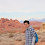## Wednesday, July 17, 2019

### Don't Fear the x, and other lessons from Shamir Secret Sharing

```f(x) = 1822 + 3x + 19x2 + 2x3
```

Fact 1. This is considered a 3rd degree polynomial because the largest exponent value is 3. While looking at this equation gives me a burst of High School Math PTSD, it need not. Upon further reflection, it's just a bit of terse code. I could program the above as:

```(define f (lambda (x)
(+ 1822
(* 3 x)
(* 19 (expt x 2))
(* 2  (expt x 3)))))
```

Because all polynomials have the same shape, it's easy to make a function that generates polynomials:

```(define (make-poly coeffs)
(lambda (x)
(let loop ((coeffs coeffs)
(i 0)
(sum 0))
(cond ((null? coeffs) sum)
(else
(loop (cdr coeffs)
(+ i 1)
(+ sum (* (car coeffs) (^ x i)))))))))
```

With this 'make' function, I can replace the above code with the following:

```(define f (make-poly '(1822 3 19 2)))
```

I can call f with as many values of x as I wish:

```> (for-each (lambda (x) (show (cons x (f x)))) (range 0 10))
((0 . 1822))
((1 . 1846))
((2 . 1920))
((3 . 2056))
((4 . 2266))
((5 . 2562))
((6 . 2956))
((7 . 3460))
((8 . 4086))
((9 . 4846))
((10 . 5752))
```

Fact 2. Evaluating a polynomial at x = 0 always returns the value of the first constant term. We see this above, as 0 maps to 1822. This will continue to hold true no matter how ugly and complex the polynomial is.

Fact 3. Using Lagrange Interpolating polynomials we can use degree + 1 values of a polynomial to construct a function which will return values equivalent to the original polynomial. That is, if we feed any four values above into make-lagr-poly we end up with a new function fg which computes the same values as f.

```(define fg (make-lagr-poly '((3 . 2056)
(6 . 2956)
(7 . 3460)
(10 . 5752))))

> (for-each (lambda (x) (show (cons x (fg x)))) (range 0 10))
((0 . 1822))
((1 . 1846))
((2 . 1920))
((3 . 2056))
((4 . 2266))
((5 . 2562))
((6 . 2956))
((7 . 3460))
((8 . 4086))
((9 . 4846))
((10 . 5752))
```

The how and why of Lagrange polynomials will have to wait for another blog post. But for now, trust me that this bit of math-magic works.

Cryptographer Adi Shamir combined the above facts to create something quite useful: a way to securely share a secret among a group.

Consider this problem:

You need to distribute a vault code to bank executives, however you'd like to avoid a number of pitfalls. For one, you want to make sure that a single lost or stolen code doesn't allow an attacker into the vault. You'd also like to insure that at least 4 executives need to agree on the requirement of opening the vault before it can be accessed. This reduces the chances that a small number of executives will coordinate to steal from the vault.

Shamir used the above math to create Shamir Secret Sharing. Here's how it works. First, note your secret, which in this case is the vault code. We'll use 12345 as our code. Then decide on your threshold, that is the number of executives that are required before the secret can be revealed. We'll use 4 as suggested above. Finally, decide on how many shares you want to give out. Let's assume there are 10 executives, so we'll generate 10 shares.

Step one is to form a polynomial of threshold - 1 degree, where the first term is the secret:

```;; values 4, 19 and 103 are random numbers, and should be
;; generated in a secure and truly random way.
(define secret (make-poly '(12345 4 19 103)))
```

Step two is to generate the 10 shares, one for each executive:

```> (for-each (lambda (x) (show (cons x (secret x)))) (range 1 10))
((1 . 12471))
((2 . 13253))
((3 . 15309))
((4 . 19257))
((5 . 25715))
((6 . 35301))
((7 . 48633))
((8 . 66329))
((9 . 89007))
((10 . 117285))
```

Note how we start x at 1 and not 0. Calling this function with 0 reveals our secret.

We give each pair of numbers to an executive and instruct them to keep the pair private. If an attacker obtains less than 4 shares, then the secret remains safe and no clues about the it are revealed.

When the vault needs to be opened, 4 executives pool their shares to calculate a function equivalent to the original polynomial:

```(define soln (make-lagr-poly '((1 . 12471)
(5 .  25715)
(9 . 89007)
(10 . 117285))))
```

Finally, to derive the secret we pass 0 to the solution function:

```> (soln 0)
12345
```

While the above implementation captures the essence of Shamir Secret Sharing, it's missing an important step to make it truly secure. I may cover that in a future post.

I had two takeaways from my experience implementing Shamir Secret Sharing. First, it's fascinating to see how mathematical properties can be combined to solve everyday problems

And Second, I found the math behind this scheme to be initially quite overwhelming. I struggled to both understand the mathematical notation, as well as how to convert it to code. And then it hit me: I've got a programming language that let's me directly implement polynomial and Lagrange interpolations functions; I don't need to think of them as purely symbolic expressions like so many of the examples on the web do. Putting this in terms of code and not math made all the difference for me.

What a joy it was to untangle what initially appeared to be so complex.

Find my implementation of Shamir Secret Sharing here. Find the original paper by Adi Shamir here. Enjoy!

1.2.